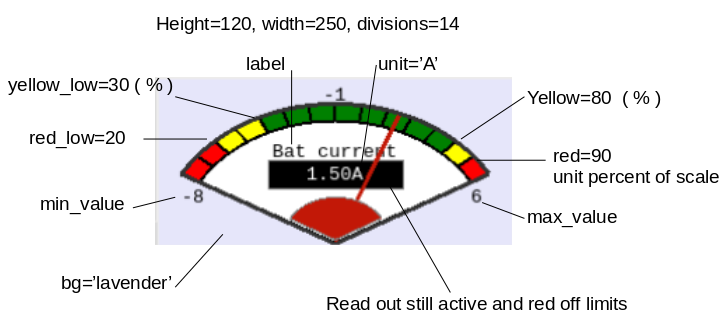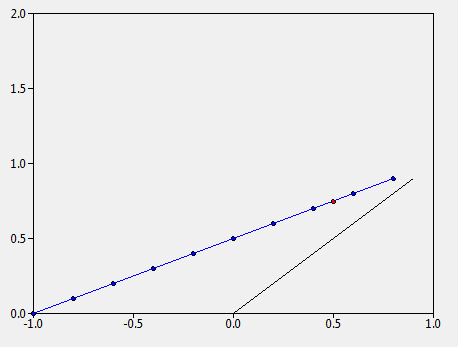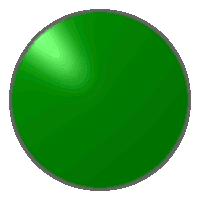# Canvas Widgets¶

These widgets provide visual feedback to the user using the canvas.

## RotaryScale¶class canvas.RotaryScale(parent, max_value: (<class 'float'>, <class 'int'>) = 100.0, size: (<class 'float'>, <class 'int'>) = 100, unit: str = None, img_data: str = None, needle_color='blue', needle_thickness=0, **options)

Shows a rotary scale, much like a speedometer.:

rs = tk_tools.RotaryScale(root, max_value=100.0, size=100, unit='km/h')
rs.grid(row=0, column=0)

rs.set_value(10)

Parameters: parent – tkinter parent frame max_value – the value corresponding to the maximum value on the scale size – the size in pixels options – the frame options
set_value(number: (<class 'float'>, <class 'int'>))

Sets the value of the graphic

Parameters: number – the number (must be between 0 and ‘max_range’ or the scale will peg the limits None

## Gauge¶class canvas.Gauge(parent, width=200, height=100, min_value=0.0, max_value=100.0, label='', unit='', divisions=8, yellow=50, red=80, yellow_low=0, red_low=0, bg='lightgrey')

Shows a gauge, much like the RotaryGauge.:

gauge = tk_tools.Gauge(root, max_value=100.0,
label='speed', unit='km/h')
gauge.grid()
gauge.set_value(10)

Parameters: parent – tkinter parent frame width – canvas width height – canvas height min_value – the minimum value max_value – the maximum value label – the label on the scale unit – the unit to show on the scale divisions – the number of divisions on the scale yellow – the beginning of the yellow (warning) zone in percent red – the beginning of the red (danger) zone in percent yellow_low – in percent warning for low values red_low – in percent if very low values are a danger bg – background

## Graph¶class canvas.Graph(parent, x_min: float, x_max: float, y_min: float, y_max: float, x_tick: float, y_tick: float, **options)

Tkinter native graph (pretty basic, but doesn’t require heavy install).:

graph = tk_tools.Graph(
parent=root,
x_min=-1.0,
x_max=1.0,
y_min=0.0,
y_max=2.0,
x_tick=0.2,
y_tick=0.2,
width=500,
height=400
)

graph.grid(row=0, column=0)

# create an initial line
line_0 = [(x/10, x/10) for x in range(10)]
graph.plot_line(line_0)

Parameters: parent – the parent frame x_min – the x minimum x_max – the x maximum y_min – the y minimum y_max – the y maximum x_tick – the ‘tick’ on the x-axis y_tick – the ‘tick’ on the y-axis options – additional valid tkinter.canvas options
draw_axes()

Removes all existing series and re-draws the axes.

Returns: None
static frange(start, stop, step, digits_to_round=3)

Works like range for doubles

Parameters: start – starting value stop – ending value step – the increment_value digits_to_round – the digits to which to round (makes floating-point numbers much easier to work with) generator
plot_line(points: list, color='black', point_visibility=False)

Plot a line of points

Parameters: points – a list of tuples, each tuple containing an (x, y) point color – the color of the line point_visibility – True if the points should be individually visible None
plot_point(x, y, visible=True, color='black', size=5)

Places a single point on the grid

Parameters: x – the x coordinate y – the y coordinate visible – True if the individual point should be visible color – the color of the point size – the point size in pixels The absolute coordinates as a tuple

## LED¶class canvas.Led(parent, size=100, on_click_callback=None, toggle_on_click=False, **options)

Create an LED-like interface for the user.:

led = tk_tools.Led(root, size=50)
led.pack()

led.to_red()
led.to_green(on=True)


The user also has the option of adding an on_click_callback function. When the button is clicked, the button will change state and the on-click callback will be executed. The callback must accept a single boolean parameter, on, which indicates if the LED was just turned on or off.

Parameters: parent – the parent frame size – the size in pixels on_click_callback – a callback which accepts a boolean parameter ‘on’ options – the frame options
to_green(on: bool = False)

Change the LED to green (on or off).

Parameters: on – True or False None
to_grey(on: bool = False)

Change the LED to grey.

Parameters: on – Unused, here for API consistency with the other states None
to_red(on: bool = False)

Change the LED to red (on or off) :param on: True or False :return: None

to_yellow(on: bool = False)

Change the LED to yellow (on or off) :param on: True or False :return: None